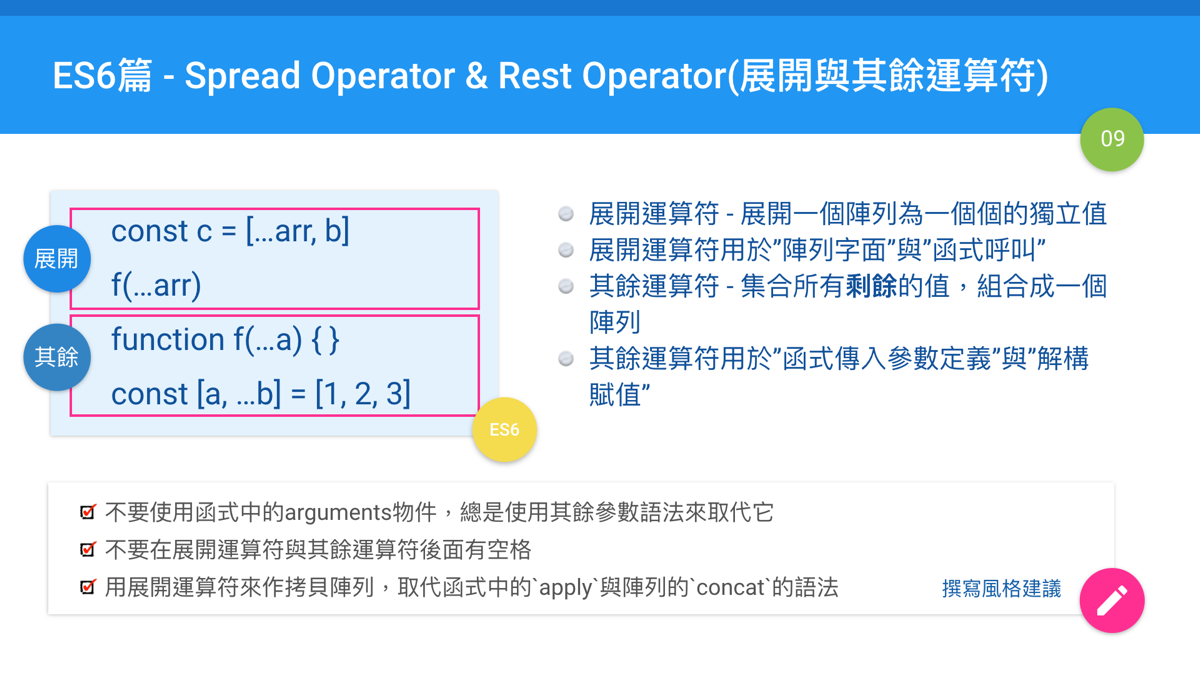# ES6篇: Spread Operator & Rest Operator(展開與其餘運算符)• 符號都是三個點(...)
• 都是在陣列值運算
• 一個是展開陣列中的值，一個是集合其餘的值成為陣列

``````const params = [ "hello", true, 7 ]
const other = [ 1, 2, ...params ] // [ 1, 2, "hello", true, 7 ]
``````

``````const arr = [1,2,3]
const arr2 = [...arr]

arr2.push(4) //不會影響到arr
``````

``````function sum(a, b, c) {
return a + b + c
}
const args = [1, 2, 3]
sum(…args) // 6
``````

``````function sum(a, b, c) {
return a + b + c;
}

var args = [1, 2, 3];
sum.apply(undefined, args) ;// 6
``````

``````const aString = "foo"
const chars = [ ...aString ] // [ "f", "o", "o" ]
``````

``````function aFunc(x){
console.log(arguments)
console.log(Array.isArray(arguments))

//轉為真正的陣列
const arr = [...arguments]
console.log(arr)
console.log(Array.isArray(arr))
}

aFunc(1)
``````

## 其餘運算符(Rest Operator)

### 其餘參數(Rest parameters)

``````function sum(…numbers) {
const result = 0

numbers.forEach(function (number) {
result += number
})

return result
}

sum(1) // 1
sum(1, 2, 3, 4, 5) // 15
``````

``````function aFunc(x, ...y){
console.log('x =', x,  ', y = ' , y)
}

aFunc(1,2,3) //x = 1, y = [2, 3]
aFunc() //x = undefined, y = []
``````

### 解構賦值(destructuring)時

``````const [x, y, z] = [1, 2, 3]

console.log(x) //1
``````

``````const [x, ...y] = [1, 2, 3]

console.log(x) //1
console.log(y) //[2,3]
``````

``````const [x, y, ...z] = 

console.log(x) //1
console.log(y) //undefined
console.log(z) //[]
``````

``````function f(...[a, b, c]) {
return a + b + c;
}

f(1)          // NaN (b and c are undefined)
f(1, 2, 3)    // 6
f(1, 2, 3, 4) // 6 (the fourth parameter is not destructured)
``````

``````// Rest Properties
let { x, y, ...z } = { x: 1, y: 2, a: 3, b: 4 }
console.log(x) // 1
console.log(y) // 2
console.log(z) // { a: 3, b: 4 }

let n = { x, y, ...z }
console.log(n) // { x: 1, y: 2, a: 3, b: 4 }
``````

## 撰寫風格建議

• 不要使用函式中的arguments物件，總是使用其餘參數語法來取代它。(Airbnb 7.6, Google 5.5.5.2, eslint: prefer-rest-params).
• 用展開運算符的語法來作拷貝陣列。(Airbnb 4.3)
• 用展開運算符的語法來取代函式中的`apply`的語法，作不定個數傳入參數的函式呼叫。(Airbnb 7.14, eslint: prefer-spread)
• 用展開運算符的語法來取代`slice``concat`方法的語法。(Google 5.2.5)
• 優先使用物件展開運算符(object spread operator)的語法取代Object.assign，來作物件的淺拷貝。(Airbnb 3.8) (ES7+標準)

## 結論

• 展開運算符: 用在陣列的字面文字定義裡面(例如`[1, ...b]`)，或是函式呼叫時(例如`func(...args)`)
• 其餘運算符: 用在函式的定義，裡面的傳入參數名稱定義時(例如`function func(x, ...y)`)。或是在解構賦值時(例如`const [x, ...y] = [1,2,3]`)